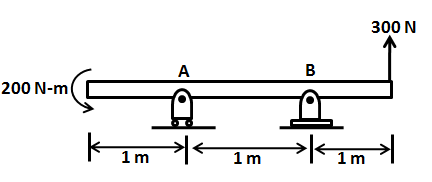# Consider the beam shown below. First represent the loads (the 300- N force and the 200 N- m...

## Question:

Consider the beam shown below. First represent the loads (the 300- N force and the 200 N- m couple) by a single equivalent force; then determine the reactions at A (roller joint) and B (pin joint).## Concentrated Moment :

It acts at a point on a beam and does not produces any change in the shear force diagram but is responsible for the variation of the bending moment over the beam.It produces instantaneous variation in the moment at a point it acts.

## Answer and Explanation: 1

Single equivalent force = 300 KN upwards with 200 KN.m concentrated anticlockwise moment .

Considering the equilibrium of the beam, the reaction at the supports :

{eq}\sum V= 0 \\ R_{A}+R_{B}+300=0 \\ \sum M_{B}=0 \\ 200-(R_{A}\times 1)+(300\times 1)=0 \\ R_{A}=500 \ KN \\ R_{B}=-300-(500) \\ =-800 \ KN \\ {/eq}

#### Learn more about this topic:External Force: Definition & Examples

from

Chapter 1 / Lesson 22
32K

In this lesson, we are going to learn about external forces and their effects on the body to which they are applied. We are also going to distinguish external forces from internal forces.